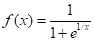Chapter 2.2, Problem 13E### Single Variable Calculus: Early Tr...

8th Edition
James Stewart
ISBN: 9781305270343

#### Solutions

Chapter
Section### Single Variable Calculus: Early Tr...

8th Edition
James Stewart
ISBN: 9781305270343
Textbook Problem

# Use the graph of the function f to state the value of each limit, if it exists. If it does not exist, explain why.(a) lim x → 0 − f ( x ) ​ (b) lim x → 0 + f ( x ) (c) lim x → 0 f ( x )(a)

To determine

To estimate: The value of limx011+e1x from the graph of f(x)=11+e1x.

Explanation

Using the graphing calculator, the graph of the function f(x)=11+e1x is drawn and shown below in Figure 1.

(b)

To determine

To estimate: The value of limx0+11+e1x from the graph of f(x)=11+e1x.

(c)

To determine

To estimate: The value of limx011+e1x from the graph of f(x)=11+e1x.

### Still sussing out bartleby?

Check out a sample textbook solution.

See a sample solution

#### The Solution to Your Study Problems

Bartleby provides explanations to thousands of textbook problems written by our experts, many with advanced degrees!

Get Started

#### Find more solutions based on key concepts